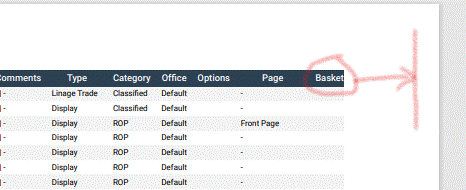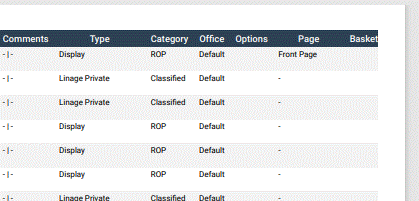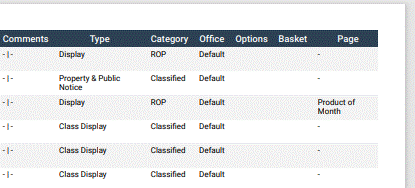# How to change page margins and still have table stretch to fit?

I am customizing PDF output from an instance of DataTables in my data extraction app. I have been able to narrow the page margins, but the table doesn't seem to stretch to fit the newly available page space due to the decreased page margins but rather stays at the same width as the available space on the page with standard page margins. I am assuming to max/min column width and overall `_maxWidth` properties are calculated before the customize function is applied. How can I make the table stretch to fit the newly manipulated page body width?

Here is my PDF customize function:

``````{
extend: 'pdf',
footer: true,
orientation: 'landscape',
title: 'App data PDF',
pageSize: 'A4',
customize: function(doc) {
doc.pageMargins = [ 20, 20, 20, 20 ];
doc.defaultStyle.fontSize = 6;
doc.styles.tableFooter.fontSize = 7;
doc.content._maxWidth = 760;             // doesn't seem to change it, last col still truncated
var rowCount = doc.content.table.body.length;
for (c = 1; c < doc.content.table.body.length; c++) {     // right align headers for numeric cols
if (["Revenue","Vol.","Yield"].includes(doc.content.table.body[c].text)) {
doc.content.table.body[c].alignment = 'right';
};
}
for (i = 1; i < rowCount; i++) {
for (c = 1; c < doc.content.table.body[i].length; c++) { // right align numeric cols
if (["Revenue","Vol.","Yield"].includes(doc.content.table.body[c].text)) {
doc.content.table.body[i][c].alignment = 'right';
};
}
}
}
},
``````## Replies

``````doc.content._maxWidth = 760;
``````

try setting `doc.content.table.widths` . Hopefully that'll do the trick,

Colin

• edited April 24

Thank you for the advice. It has improved a bit. The table now uses the full width, but the last column is still truncated slightly, however, this is an improvement.

I set each width to `"auto"` as setting them to `"*"` made each column the same width and it definitely didn't fit the page. I can see this is largely a pdfMake issue and so I'll try focussing my efforts there and will report back if I find a solution.

The extra code I used in the `customize` function was:

``````    var tbl = \$('#app-data-table');
var colCount = new Array();
\$(tbl).find('tbody tr:first-child td, tbody tr:first-child th').each(function(){
if(\$(this).attr('colspan')){
for(var i=1;i<=\$(this).attr('colspan');\$i++){
colCount.push('auto');
}
}else{ colCount.push('auto'); }
});
doc.content.table.widths = colCount;
``````• Actually, I have just discovered something. It seems my final column with no data wasn't actually being truncated, it simply looked that way due to minimal gutters (padding/margin) between columns.

I swapped the last two columns around and it actually looks right now, so your suggested change with my tweak as shown above is a working solution... thank you!• For the sake of completeness for anyone reading this with the same issue, here is my the full `customize` code of my final solution:

``````customize: function(doc) {
doc.pageMargins = [ 20, 20, 20, 20 ];
doc.defaultStyle.fontSize = 6;
doc.styles.tableFooter.fontSize = 7;

var rowCount = doc.content.table.body.length;
for (c = 1; c < doc.content.table.body.length; c++) {     // right align headers for numeric cols
if (["Revenue","Vol.","Yield"].includes(doc.content.table.body[c].text)) {
doc.content.table.body[c].alignment = 'right';
};
}
for (i = 1; i < rowCount; i++) {
for (c = 1; c < doc.content.table.body[i].length; c++) { // right align numeric cols
if (["Revenue","Vol.","Yield"].includes(doc.content.table.body[c].text)) {
doc.content.table.body[i][c].alignment = 'right';
};
}
}
var tbl = \$('#app-data-table');
var colCount = new Array();
\$(tbl).find('tbody tr:first-child td, tbody tr:first-child th').each(function(){
if(\$(this).attr('colspan')){
for(var i=1;i<=\$(this).attr('colspan');\$i++){
colCount.push('auto');
}
}else{ colCount.push('auto'); }
});
doc.content.table.widths = colCount;
}

``````